Check Full Chapter Explained - Continuity and Differentiability - Continuity and Differentiability Class 12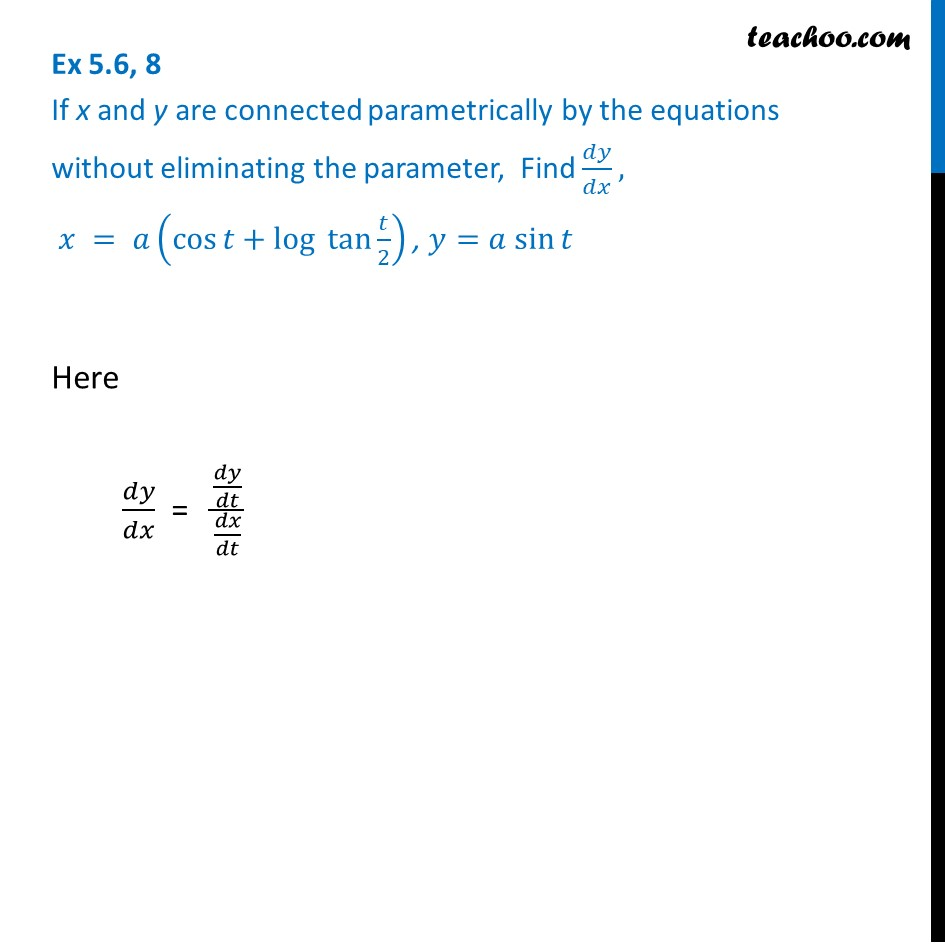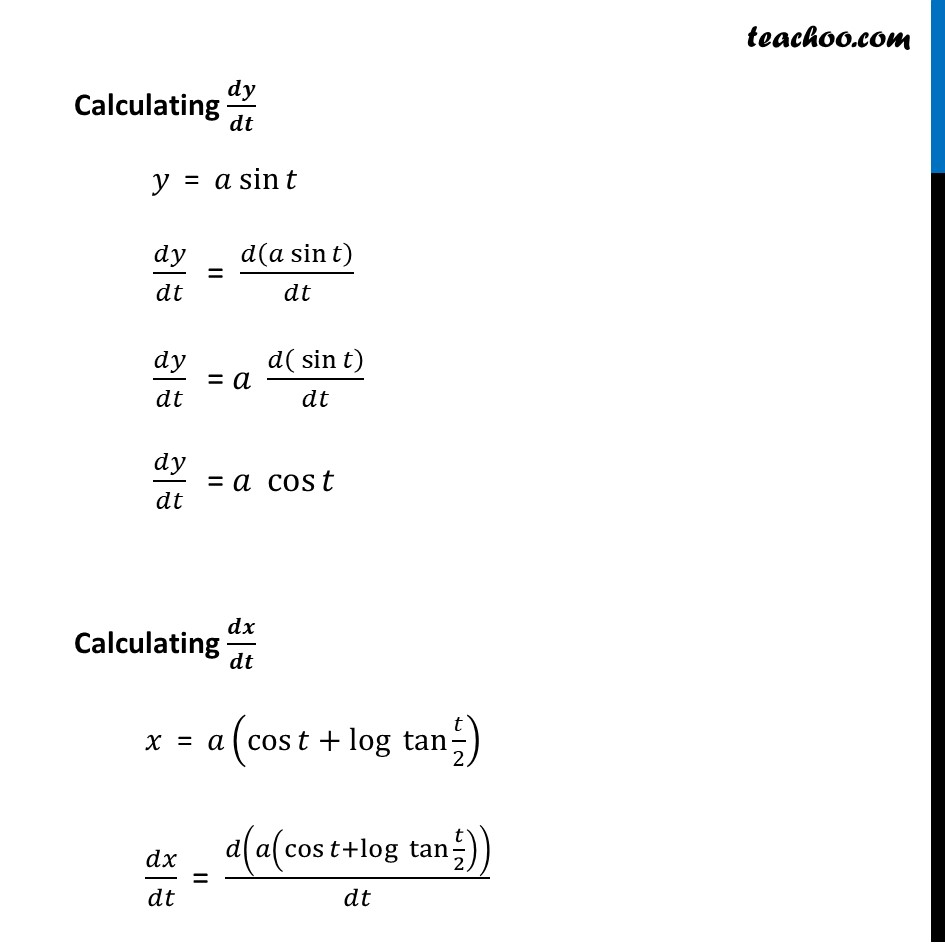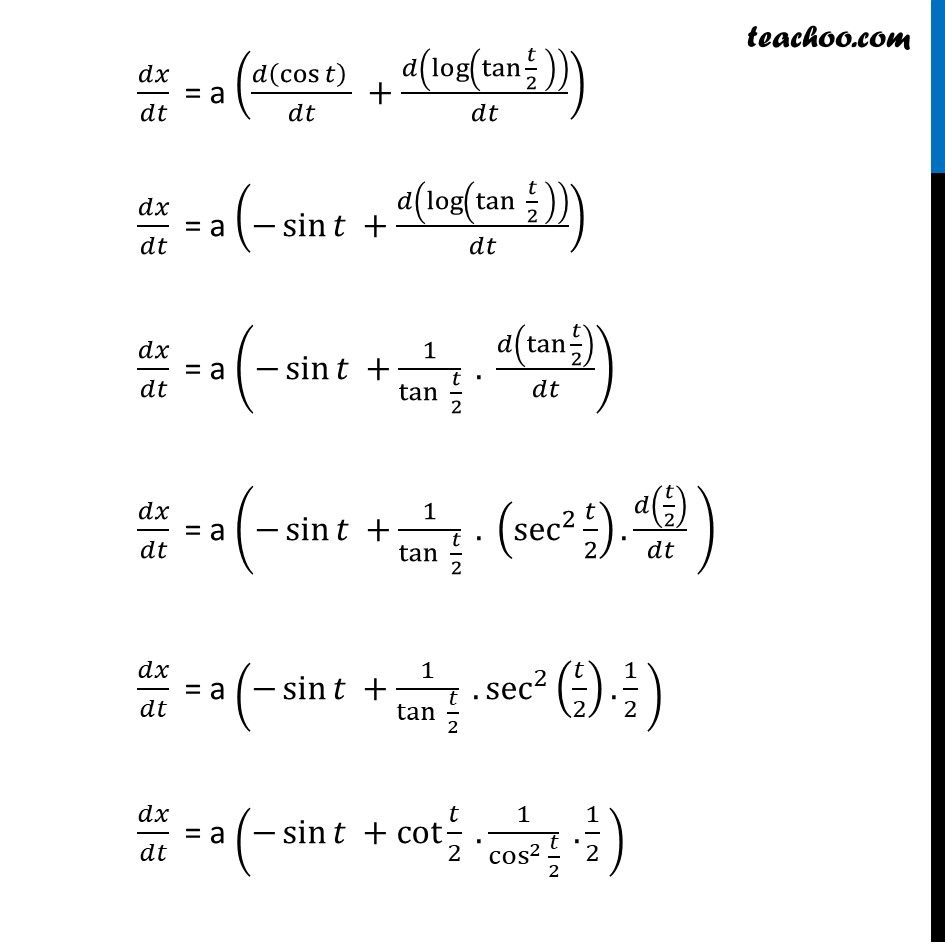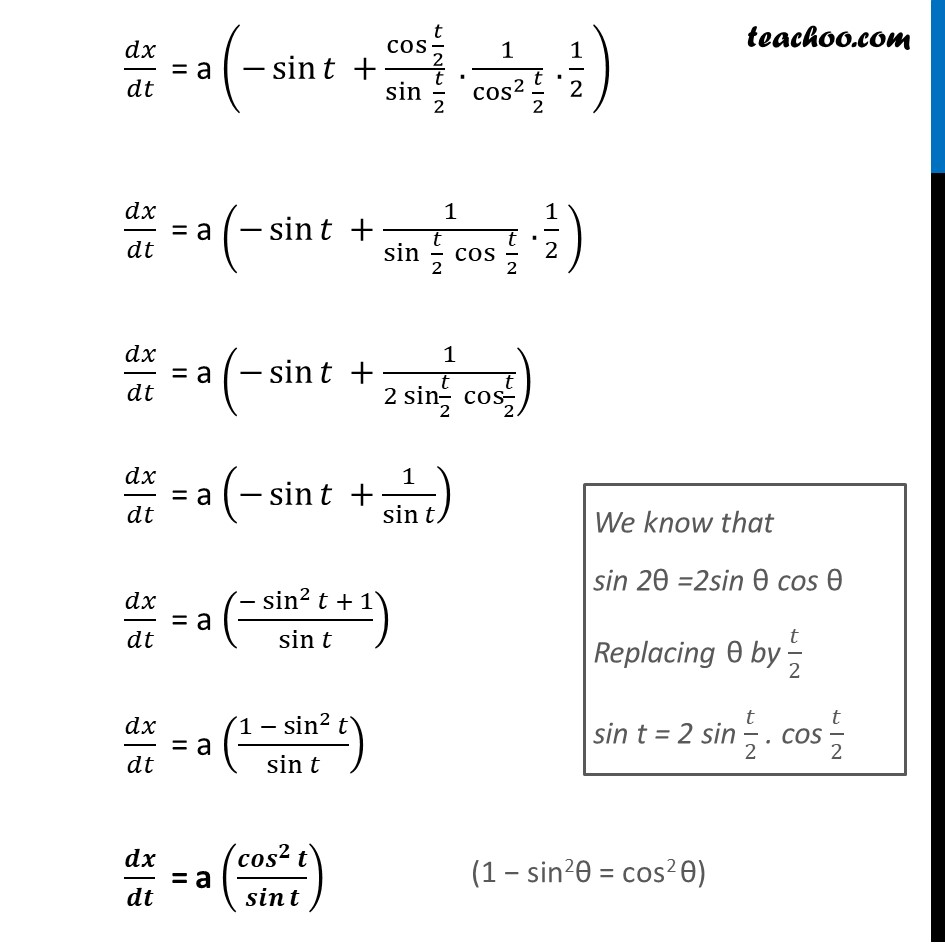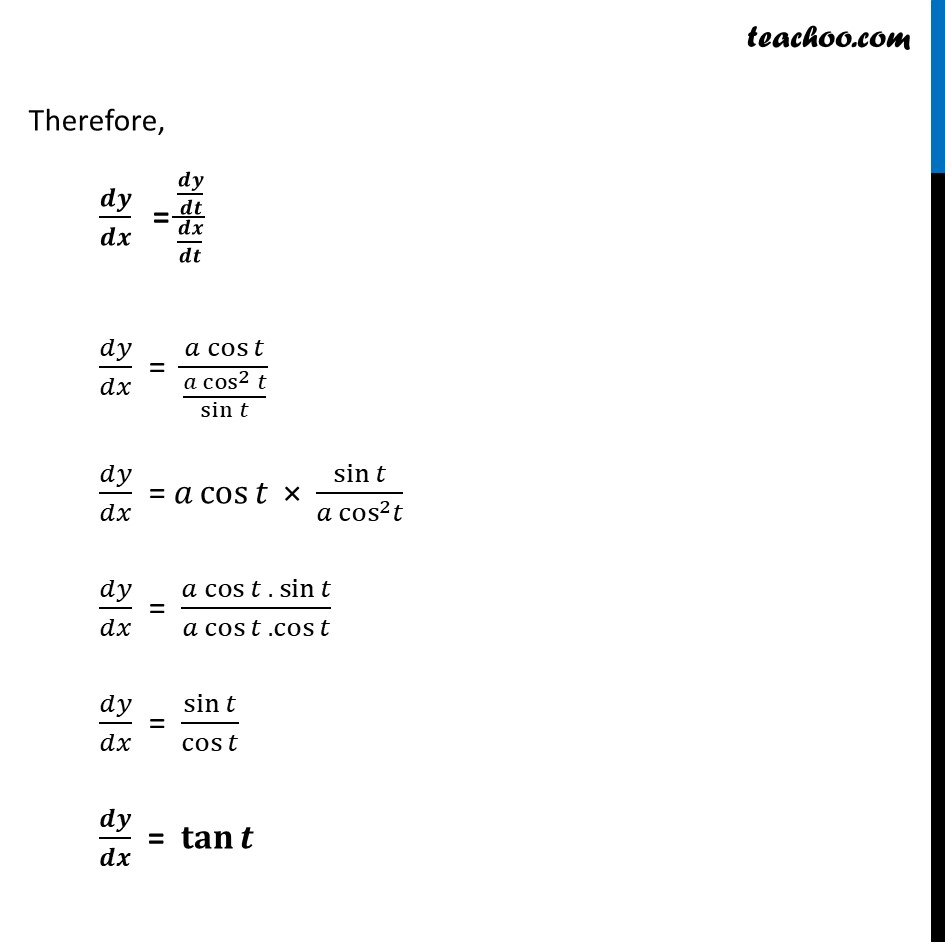1. Chapter 5 Class 12 Continuity and Differentiability
2. Serial order wise
3. Ex 5.6

Transcript

Ex 5.6, 8 If x and y are connected parametrically by the equations without eliminating the parameter, Find 𝑑𝑦/𝑑𝑥, 𝑥 = 𝑎(cos⁡〖𝑡+log⁡〖 tan〗⁡〖𝑡/2〗 〗 ) , 𝑦=𝑎 sin⁡𝑡Here 𝑑𝑦/𝑑𝑥 = (𝑑𝑦/𝑑𝑡)/(𝑑𝑥/𝑑𝑡) Calculating 𝒅𝒚/𝒅𝒕 𝑦 = 𝑎 sin⁡𝑡 𝑑𝑦/𝑑𝑡 " " = 𝑑(𝑎 sin⁡𝑡)/𝑑𝑡 𝑑𝑦/𝑑𝑡 " " = 𝑎 𝑑( sin⁡𝑡)/𝑑𝑡 𝑑𝑦/𝑑𝑡 " " = 𝑎 cos⁡𝑡 Calculating 𝒅𝒙/𝒅𝒕 𝑥 = 𝑎(cos⁡〖𝑡+log⁡〖 tan〗⁡〖𝑡/2〗 〗 ) 𝑑𝑥/𝑑𝑡 = 𝑑(𝑎(cos⁡〖𝑡+log⁡〖 tan 〗⁡〖𝑡/2〗 〗 ))/𝑑𝑡 𝑑𝑥/𝑑𝑡 = a ((𝑑(cos⁡𝑡 ) )/𝑑𝑡 +𝑑(log⁡(〖tan 〗⁡〖𝑡/2〗 ) )/𝑑𝑡) 𝑑𝑥/𝑑𝑡 = a (−sin⁡𝑡 +𝑑(log⁡(tan⁡〖 𝑡/2〗 ) )/𝑑𝑡) 𝑑𝑥/𝑑𝑡 = a (−sin⁡𝑡 +1/tan⁡〖 𝑡/2〗 . 𝑑(〖tan 〗⁡〖𝑡/2〗 )/𝑑𝑡) 𝑑𝑥/𝑑𝑡 = a (−sin⁡𝑡 +1/tan⁡〖 𝑡/2〗 . (sec^2⁡〖𝑡/2〗 ).𝑑(𝑡/2)/𝑑𝑡 ) 𝑑𝑥/𝑑𝑡 = a (−sin⁡𝑡 +1/tan⁡〖 𝑡/2〗 . sec^2 (𝑡/2). 1/2 ) 𝑑𝑥/𝑑𝑡 = a (−sin⁡𝑡 +cot⁡〖𝑡/2〗 . 1/(cos^2 𝑡/2) . 1/2 ) 𝑑𝑥/𝑑𝑡 = a (−sin⁡𝑡 +〖cos 〗⁡〖𝑡/2〗/sin⁡〖 𝑡/2〗 . 1/(cos^2 𝑡/2) . 1/2 ) 𝑑𝑥/𝑑𝑡 = a (−sin⁡𝑡 +1/(sin⁡〖 𝑡/2〗 cos⁡〖 𝑡/2〗 ) . 1/2 ) 𝑑𝑥/𝑑𝑡 = a (−sin⁡𝑡 +1/(2 sin⁡〖𝑡/2〗 cos⁡〖𝑡/2〗 )) 𝑑𝑥/𝑑𝑡 = a (−sin⁡𝑡 +1/sin⁡𝑡 ) 𝑑𝑥/𝑑𝑡 = a ((−sin^2⁡𝑡 + 1)/sin⁡𝑡 ) 𝑑𝑥/𝑑𝑡 = a ((1 − sin^2⁡𝑡)/sin⁡𝑡 ) 𝒅𝒙/𝒅𝒕 = a (〖𝒄𝒐𝒔〗^𝟐⁡𝒕/𝒔𝒊𝒏⁡𝒕 ) We know that sin 2θ =2sin θ cos θ Replacing θ by 𝑡/2 sin t = 2 sin 𝑡/2 . cos 𝑡/2 Therefore, 𝒅𝒚/𝒅𝒙 " =" (𝒅𝒚/𝒅𝒕)/(𝒅𝒙/𝒅𝒕) 𝑑𝑦/𝑑𝑥 = (𝑎 cos⁡𝑡)/(〖𝑎 cos^2〗⁡𝑡/sin⁡𝑡 ) 𝑑𝑦/𝑑𝑥 = 𝑎 cos⁡𝑡 × sin⁡𝑡/(𝑎 cos^2 𝑡) 𝑑𝑦/𝑑𝑥 = (𝑎 cos⁡𝑡 . sin⁡𝑡)/(𝑎 cos⁡𝑡 .cos⁡𝑡 ) 𝑑𝑦/𝑑𝑥 = sin⁡𝑡/cos⁡𝑡 𝒅𝒚/𝒅𝒙 = 𝐭𝐚𝐧⁡𝒕

Ex 5.6

About the AuthorDavneet Singh
Davneet Singh is a graduate from Indian Institute of Technology, Kanpur. He has been teaching from the past 9 years. He provides courses for Maths and Science at Teachoo.